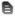# Description and Tips for the Mathematics Section of the GEDThe mathematics section is one of four sections of the GED exam. This article presents information that will help you while taking this portion of the exam.
View available schools

### The GED Mathematics Section

The General Education Development (GED) exam is used by many states to offer a credential equivalent to a high school diploma. Mathematical reasoning is one of four subject area tests that must be passed in order to earn the GED credential.

The following details about the math portion of the test reflect substantial changes that were made to the GED in 2014. The test is now taken on a computer at an official testing site, so questions can take various forms such as multiple choice, fill-in-the-blank and drag-and-drop.

A formula sheet is provided so that test-takers can focus on applying principles rather than memorizing formulas, and a calculator is permitted after the first five test questions are completed. A period of 115 minutes is allotted for the math exam.

#### Mathematics Test Specifics

The GED Testing Service has designed the test to cover procedural skills, problem solving and math fluency. The test is roughly split in half between quantitative problem solving and algebraic problem solving.

The following skills and concepts are listed by the GED Testing Service as being the focus of the math portion of the exam:

• Apply number sense concepts with rational numbers
• Perform operations on rational number
• Solve problems with rational numbers, ratios, proportions or percents (focus is on real-world examples)
• Use Pythagorean Theorem
• Compute surface area and volume (formulas are provided)
• Evaluate expressions by substituting integers for unknown quantities

#### Mathematics Test Tips

The Independent Learning Centre (ILC), www.ilc.org, recommends that you familiarize yourself with the TI-30XS calculator's operations before taking the test; whether you use a handheld version or the on-screen option provided with the test, that is the only calculator allowed.

Becoming familiar with what is included on the mathematics formulas page in advance of the test is also smart, so that you will be able to find this information quickly while taking the test. You will not need to memorize the formulas.

ILC has several good points about information given in the problem: Some questions provide you with more information than you need in order to reach the answer. Use only the data relevant to the particular question. Also, some multiple-choice questions may contain insufficient information to answer the question. In that case, the correct response is 'Not enough information is given.' Last, in diagrams, do not assume anything. For example, just because lines look parallel or perpendicular doesn't mean they are. Only if the problem explicitly states, using symbols or words, that the diagram is parallel or perpendicular should you take them to be this way.

Be aware that whatever you get for an answer, just take a second or two to see if your answer if logical; look at the initial question and then glance at your answer given the parameters of the problem. ILC gives the following example: If your calculations indicate that a 1 lb. bag of carrots will cost \$25, you should check your work for errors because \$25 is an unreasonable price for such an item.

Above all, use your own experience to solve problems. Since the context of the problems are usually realistic, think, 'what would I do in this situation?' ILC gives another good example: In a test question requiring you to compute weekly earnings, ask yourself, 'How would I calculate my weekly earnings?' This is a likely scenario and one that you would probably want to know more than once in your life.

## Popular Schools

• ### Online Programs Available 1. Penn Foster High School

#### Program Options

High School Diploma
• HS Diploma
• HS Diploma
• Cambridge, MA

• Durham, NC

### Duke University

• Notre Dame, IN

### University of Notre Dame

• Nashville, TN

### Vanderbilt University

• Gainesville, FL

• Ithaca, NY

• Stanford, CA

• Boston, MA

• HS Diploma